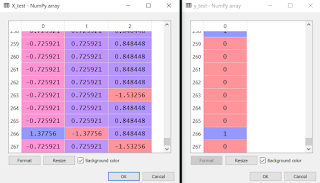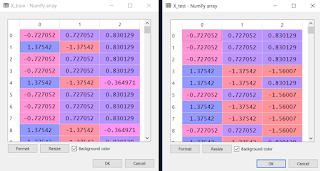### Problem Statement: Predict whether or not a passenger survived during Titanic Sinking

Variables: PassengerID, Survived, Pclass, Name, Sex, Age, Fare

We are going to use two variables i.e. Pclass and sex of the titanic passsengers to predict whether they survived or not

Independent Variables : Pclass, Sex
Dependent Variable : Survived

# Encoding categorical data
from sklearn.preprocessing import LabelEncoder, OneHotEncoder
labelencoder_X = LabelEncoder()
X[:, 1] = labelencoder_X.fit_transform(X[:, 1])
onehotencoder = OneHotEncoder(categorical_features = )
X = onehotencoder.fit_transform(X).toarray()

We have encoded the variable "Sex" in X which had two categorical values i.e. male and female. Hence, we've got 2 different columns. Third column in the picture below is for the variable "Pclass".# Splitting the dataset into the Training set and Test set
from sklearn.cross_validation import train_test_split
X_train, X_test, y_train, y_test = train_test_split(X, y, test_size = 0.30, random_state = 0)# Feature Scaling
from sklearn.preprocessing import StandardScaler
sc = StandardScaler()
X_train = sc.fit_transform(X_train)
X_test = sc.transform(X_test)

We did feature scaling as we want to obtain an accurate prediction of whether a passenger survived the sinking of titanic or not. Also, Feature Scaling is a must do preprocessing step when the algorithm is based on Euclidean Distance.# Fitting Naive Bayes to the Training set
from sklearn.naive_bayes import GaussianNB
classifier = GaussianNB()
classifier.fit(X_train, y_train)

We created an object 'classifier' of class 'GaussianNB' and fitted it into our training set.

# Predicting the Test set results
y_pred = classifier.predict(X_test)# Making the Confusion Matrix
from sklearn.metrics import confusion_matrix
cm = confusion_matrix(y_test, y_pred)

Confusion Matrix helps to know how good our model is predicting. In other words, we will assess how correctly our Logistic Regression Model has learned the correlations from the training set to make accurate predictions on the test set.Here, the diagonal with 140 and 71 shows the correct predictions and the diagonal 29 and 28 shows the incorrect predictions.
So, 140 + 71 = 211 are the total number of correct predictions out of 268 instances (in y_test)
Hence, our model showed 78.7% accuracy.